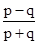Subscribe Now

SSC CPO : Quantitative Aptitude Quiz | 25 - 04 - 18

Mahendra Guru

In SSC exam, quantitative Aptitude section is more scoring and easy, if you know the shorts tricks and formulas of all the topics. So, it is important to know the basic concepts of all the topics so you can apply the short tricks and solve the question with the new concept sin lesser time while giving the quiz. It will help you to score more marks from this section in less time period. Quantitative Aptitude section basically measures your mathematical and calculation approach of solving the question. SSC Quiz of quantitative Aptitude section helps you to analyse your preparation level for upcoming SSC examination. Mahendra Guru provides you Quantitative Aptitude Quiz for SSC examination based on the latest pattern so that you can practice on regular basis. It will definitely help you to score good marks in the exam. It is the most important section for all the govt exams like Insurance, SSC-MTS, SSC CPO , CGL, CHSL, State Level, and other Competitive exams.

Mahendra Guru also provides you important notes and study material for all subject and test through its website, Mahendra Guru App and YouTube channel apart from it Speed Test Portal. Most of these preparation products are also available for purchase on my shop. You can also visit Mahendras.org to get more information about our endeavour for your success. You can also study in details through our E-Mahendras Facebook and Mahendra Guru YouTube channel of Quantitative Aptitude.

Q.1. The simple interest on an amount of Rs.27500 at the end of three years is Rs.10230. What would be the approximate compound interest accrued on the same amount at the same rate in the same period?
(A) Rs./रु.11900
(B) Rs./रु.12620
(C) Rs./रु.10950
(D) Rs./रु.11550

Q.2. 1.236 × 1015 – 5.23 × 1014 is equal to:
1.236 × 1015 – 5.23 × 1014 बराबर है:
(A) 7.13 × 1014
(B) 7.13 × 1015
(C) 71.3 × 1014
(D) – 3. 994
Q.3. If, then the value ofis equal to:

यदि, तोका मान बराबर है:
(A) 7.826
(B) 8.994
(C) 5.59
(D) 10.062

Q.4. 96 + 7, when divided by 8, would have a remainder:

96 + 7, को जब 8 से विभाजित किया जाता है, एक शेषफल देगा:
(A) 7
(B) 6
(C) 5
(D) 0

Q.5. The unit digit of (795 – 358) is:

(795 – 358) का इकाई का अंक है:
(A) 8
(B) Lies between 6 and 10/6 और 10 के मध्य स्थित है
(C) 6
(D) Lies between 3 and 6/3 और 6 के मध्य स्थित है

Q.6. Ifthen find the value ofयदितोका मान ज्ञात कीजिये |
(A) 18
(B) 36
(C) 49
(D) 343

Q.7.when simplified is:जब सरलीकृत होगा तो:
(A) 1 – 2(2n)
(B) 2n + 3 –(C)(D)Q.8. The third proportional between (p2 – q2) and (p + q) 2 is:

(p2 – q2) और (p + q) 2 का तृतीयानुपाती है:
(A)(B)(C)(D)Q.9. The average marks of 65 students in a class was calculated as 150. It was later realized that the marks of one of the student was calculated as 142, whereas his actual marks were 152. What is the actual average marks of the group of 65 students? (Rounded off two digits after decimal)

कक्षा में 65 छात्रों के औसत अंकों की गणना 150 की गई थी। बाद में यह ज्ञात किया गया कि एक छात्र के अंक की गणना 142 की गयी थी, जबकि उसके वास्तविक अंक 152 थे। 65 छात्रों के समूह के वास्तविक औसत अंक क्या हैं? (दशमलव के बाद दो अंक तक)
(A) 151.25
(B) 150.15
(C) 151.10
(D) 150.19
Q.10. A can dowork in 5 days. B can doof same work in 9 days and C can doof that work in 8 days. In how many days can three of them together do the work?
A किसी कार्य केभाग को 5 दिन में पूरा कर सकता है, B उसी कार्य केभाग को 9 दिन में पूरा कर सकता है, और C उसी कार्य केभाग को 8 दिन में पूरा कर सकता है | कितने दिनों में वे तीनों एकसाथ मिलकर कार्य को पूरा करते हैं?
(A) 5 days/दिन
(B) 4days/दिन
(C) 3 days/दिन
(D) 4 days/दिन

Q1. (D)R = 12.4%
C.I. == Rs./रु.11550

Q2. (A) 1.236 × 1015 – 5.23 × 1014 = 1014 (1.236 × 10 – 5. 23)
= 1014 (12.36 – 5.23) = 7.13 × 1014

Q3. (A)==== 7.826
Q4. (D) Required remainder/अभीष्ट शेषफल == 0

Q5. (D) Unit's digit in 795/795 में इकाई का अंक = 3
Unit's digit in 358/358 में इकाई का अंक = 9
Unit's digit in (795 – 358)/(795 – 358) में इकाई का अंक = 13 – 9 = 4
Lies between 3 and 6/3 और 6 के मध्य स्थित है
Q6. (A)= 18

Q7. (C)=== 2n + 1 – 2n – 2
= 2– (n + 1) =Q8. (D) Third proportional/ तृतीयानुपाती ===Q9. (B) Required correct sum of marks/अभीष्ट सही अंकों का योग
= 65 × 150 – 142 + 152 = 9760
Required average/अभीष्ट औसत == 150.15

Q10. (D) 6 ………. A ……… 10
4 ……….. B ………. 15
5 ……….. C ………. 12
(Total Work/कुल कार्य = 60)
According to the question/प्रश्नानुसार,
required time/अभीष्ट समय == 4 days/दिन